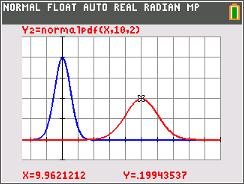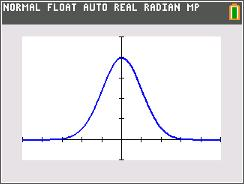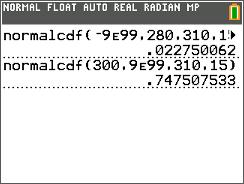# Activities

••• ##### Subject Area

• Math: Algebra II: Data Analysis

• ##### Author9-12

45 Minutes

• ##### Device
• TI-84 Plus
• TI-84 Plus Silver Edition
•TI-84 Plus C Silver Edition
•TI-84 Plus CE

## Average Orange#### Activity Overview

Students explore the area under the normal curve between various x-values and use a model to find what percent of the area lies within 1, 2, and 3 standard deviations of the mean. weights. Percentiles are defined and calculated.

#### Key Steps

•In Problem 1 and 2, students explore the normal curve. Students have a discussion about a bell curve which approximates for the binomial distribution. Students will see that the curve is symmetrical and extends to infinity in both directions. Its height is greatest in the center and decreases to the right and left, approaching (but never quite reaching) zero.

•Students manipulate the shape of a normal curve by controlling two parameters: the mean, which controls the location of the hump of the curve, and the standard deviation, which controls whether the bell is broad and flat or narrow and tall. Students will see that no matter what the mean and standard deviation, the total area under every normal curve is 1.

•Problem 3 allows students to investigate an application of the normal distribution. The problem produces a real-life variable that is normally distributed. It describes the weight of each orange in a farmer’s crop.